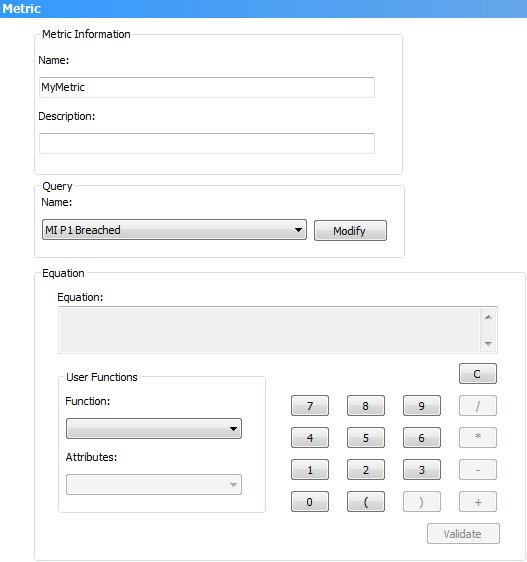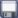### Creating an equation

Metrics provide the numeric values that are plotted on Trend charts. This number is calculated from the results of a filter query using the Metric Equation editor.

##### To create an equation:
1. Open the required Metric window.
2. Make sure that the Name and Description for the metric are completed and that the required Query Name is selected.1. Use the equation editor’s number pad and the User Functions to create the required equation.
The equation appears in the Equation box.

The functions that are available depend on the output types of the metric’s query:
Sum – adds all of the numeric values returned by the selected attributes in the query
Avg – calculates the arithmetic mean of the numeric values returned by the selected query attributes
Count – counts the total number of rows returned by the query
Min – returns the smallest value returned by the selected query attribute
Max – returns the largest value returned by the selected query attribute

You cannot edit the text in the Equation box.
To delete the current equation, click C on the equation editor’s number pad.

1. To test the syntax of your equation, click Validate.
2. When you have created the required equation and metric, clickon the toolbar.
The metric is saved.